#### 期刊菜单

Study on Relaxation Performance of Carbon Fiber Plate Based on Anchor System
DOI: 10.12677/HJCE.2022.1111139, PDF , HTML, XML, 下载: 118  浏览: 700  科研立项经费支持

Abstract: As a popular composite material in recent years, carbon fiber reinforced polymer (CFRP) sheet, apart from its reinforcement role in structural engineering, has excellent material properties compared with traditional steel cables in cable-stayed bridges and suspension bridges. Therefore, carbon fiber cables are famous in bridge field because of their low price and high engineering cost performance, and become the potential main substitute of traditional steel cables in the future. In this paper, the relaxation performance of carbon fiber composite plates based on anchorage system is mainly studied. The creep mechanical model and creep characteristics of CFRP sheets are summarized.

1. 研究背景

2. CFRP拉索蠕变特性

2.1. CFRP材料的力学性能

CFRP材料是以树脂为基材，将具有高强度的碳素纤维作为增强体通过挤压，拉拔成型，形成具有轻质高强、耐腐蚀、抗拉强度高的复合材料。碳纤维增强聚合物片材(CFRP)作为一种新型材料，在结构工程领域作为加固材料，通过对构件施加预应力大幅度提升结构的抗弯承载能力，常用于桥梁的加固工程中。碳纤维材料不仅可作为加固材料，其质量轻于钢筋、钢绞线，其抗拉强度远高于钢筋，各类材料力学性能比较见表1，比钢材更耐潮湿、腐蚀，碳纤维板为索体材料时，碳纤维拉索的耐久性与抗疲劳性能优于钢索，可显著延长结构的耐久性，大幅度减少结构的维护费用；碳纤维拉索的预应力强度高，减振性能好可适用于斜拉桥、悬索桥类的桥梁结构中。预应力张拉后的CFRP拉索的抗拉强度，强度远远高于钢筋索缆，普通碳纤维板抗拉强度为1500~2500 MPa，而普通钢筋抗拉强度为490~700 MPa，钢绞线抗拉强度为1400~1860 MPa，碳纤维板的抗拉强度约为普通钢筋的3倍，钢绞线的1.2倍，除此以外，还有一种高强度碳纤维板，抗拉强度超过3000 MPa，约为普通钢筋抗拉强度的5倍，钢绞线抗拉强度的2倍以上。Table 1. Comparison of mechanical properties between various fiber reinforcement and steel strand 

2.2. CFRP材料的蠕变机制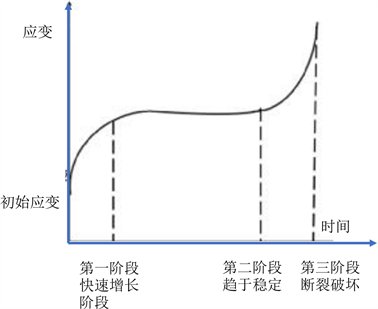Figure 1. Three stages of creep of fibrous materials

3. CFRP拉索蠕变理论

3.1. 经验方程

$\epsilon \left(t\right)={\epsilon }_{0}+{\epsilon }_{1}\left(t\right)+{\epsilon }_{2}\left(t\right)+{\epsilon }_{3}\left( t \right)$

$\epsilon \left(t\right)$ ——总蠕变量；

${\epsilon }_{0}$ ——初始弹性应变；

${\epsilon }_{1}\left(t\right)$ ——瞬间蠕变；

${\epsilon }_{2}\left(t\right)$ ——等速蠕变；

${\epsilon }_{3}\left(t\right)$ ——加速蠕变；

3.2. 流变模型

$\sigma =\kappa \epsilon$

$\sigma =\eta \epsilon =n\left(\frac{\text{d}\epsilon }{\text{d}t}\right)$

$\eta ,\epsilon$ 分别为粘滞系数和应变速率。塑性元件：塑性软件如图2(c)所示，塑性元件可以看作两个滑块之间接触摩擦，其变形特点为，当外加应力小于自身摩擦阻力时，不发生变形；当外应力超过摩擦阻力时，应力恒定的情况下发生塑性变形，初应力水平决定塑性变形，与时间无关。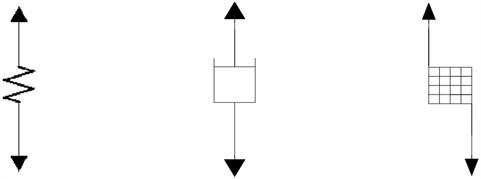(a) 弹簧 (b) 阻尼器 (c) 摩擦器

Figure 2. Basic elements of rheological model

3.2.1. Maxwell模型(串联模型)

$\epsilon ={\epsilon }_{1}+{\epsilon }_{2}$

${\epsilon }_{1}=\frac{\sigma }{k}$

$\sigma =\eta \frac{\text{d}{\epsilon }_{2}}{\text{d}t}$

$\frac{\text{d}\epsilon }{\text{d}t}=\frac{\text{d}{\epsilon }_{1}}{\text{d}t}+\frac{\text{d}{\epsilon }_{2}}{\text{d}t}$

Maxwell模型本构方程如下： $\frac{\text{d}\epsilon }{\text{d}t}=\frac{1}{k}\frac{\text{d}\sigma }{\text{d}t}+\frac{\sigma }{\eta }$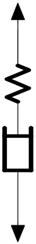Figure 3. Maxwell model

Maxwell模型能模拟出应力松弛过程，但不能模拟出聚合物的蠕变过程。

3.2.2. Kelvin模型(并联模型)

$\sigma ={\sigma }_{1}+{\sigma }_{2}$

${\sigma }_{1}=k\epsilon$

${\sigma }_{2}=\eta \frac{\text{d}\epsilon }{\text{d}t}$

Kelvin模型的本构方程如下： $\sigma =k\epsilon +\eta \frac{\text{d}\epsilon }{\text{d}t}$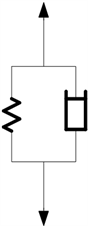Figure 4. Kelvin model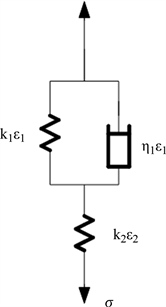Figure 5. Kelvin standard linear solid model

Kelvin模型能模拟出材料蠕变过程，但不能模拟出聚合物的应力松弛过程。

3.2.3. 标准线性固体模型

${C}_{1}=\frac{1}{{k}_{1}}$

${C}_{2}=\frac{1}{{k}_{2}}$

$\sigma ={\sigma }_{1}+{\sigma }_{2}=\frac{{\epsilon }_{1}}{{C}_{1}}+\eta \frac{\text{d}{\epsilon }_{1}}{\text{d}t}$

${\stackrel{¯}{\epsilon }}_{1}=\frac{{c}_{1}\stackrel{¯}{\sigma }}{\tau \left(s+\frac{1}{\tau }\right)}$

$\stackrel{¯}{\epsilon }=\stackrel{¯}{\epsilon }+{\stackrel{¯}{\epsilon }}_{1}=\left\{{c}_{2}+\frac{{c}_{1}}{\tau \left(s+\frac{1}{\tau }\right)}\right\}\stackrel{¯}{\sigma }$

$\frac{\epsilon \left(t\right)}{{\sigma }_{0}}={C}_{C}\left(t\right)={C}_{2}+{C}_{1}\left(1-{\text{e}}^{-\frac{t}{\tau }}\right)$

${C}_{C}$ 为蠕变柔量， ${C}_{C}\left(t\right)$ 即为与时间相关的应变变化公式。

4. 试验方案

1) CFRP板松弛加载装置设计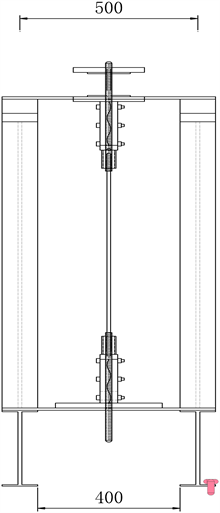Figure 6. Front elevation of test frame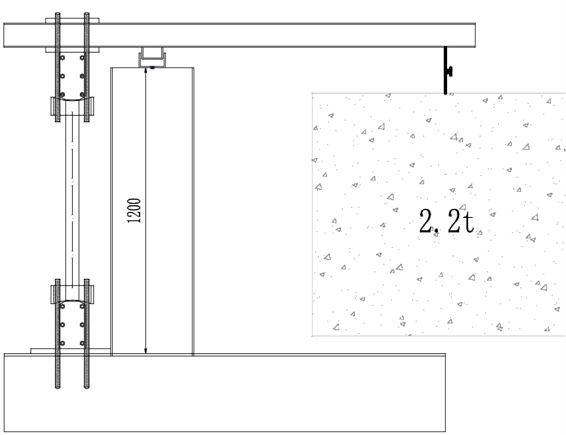Figure 7. Side elevation of test frame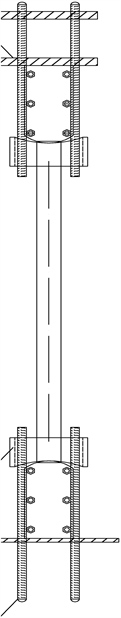Figure 8. Elevation of carbon plate

2) 实验测量装置(应变片、百分表)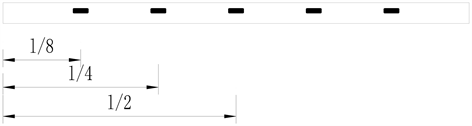Figure 9. Carbon plate strain gauge adhesion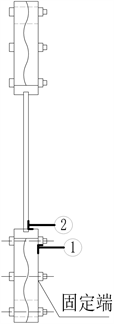Figure 10. Fixed end slip measurement

3) 碳板设计

4) 数据收集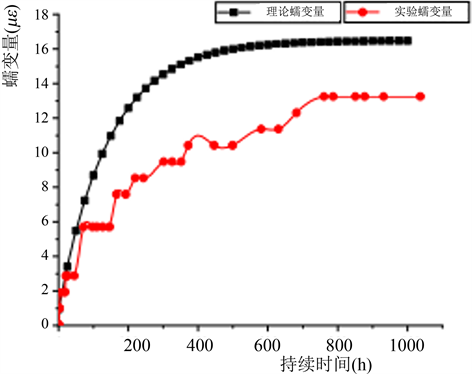Figure 11. Comparison of creep variables

5. 结论

  曾磊. 预应力CFRP板加固混凝土梁试验与理论研究[D]: [硕士学位论文]. 上海: 同济大学, 2005.  Viswanathan, C. (2001) Individual and Interactive Influence of Temperature, Stress, Physical Aging and Moisture on Creep, Creep Rupture and Fracture of Epoxy Matrix and Its Composite. The University of Manitoba, Winnipeg.  Wu, Z.S., Fahmy, M.F.M. and Wu, G. (2011) Damage-Controllable Structure Systems Using FRP Composites. Journal of Earthquake and Tsunami, 5, 241-258. https://doi.org/10.1142/S1793431111000966  Kamal, A.S.M. and Boulfiza, M. (2010) Durability of GFRP Rebars in Simulated Concrete Solutions under Accelerated Aging Conditions. Journal of Composites for Construction, 15, 473-481. https://doi.org/10.1061/(ASCE)CC.1943-5614.0000168  GB 50010-2010. 混凝土结构设计规范[S]. 北京: 中国建筑工业出版社, 2010.  井德胜, 白晓宇, 王海刚, 张明义. 玻璃纤维增强聚合物锚杆蠕变性能研究进展[J]. 复合材料科学与工程, 2022(2): 119-128.  刘鹏飞, 赵启林, 王景全. 树脂基复合材料蠕变性能研究进展[J]. 玻璃钢/复合材料, 2013(3): 109-117+12.  黎伟捷. 碳纤维复材平行板拉索及曲面板锚具性能研究[D]: [硕士学位论文]. 北京: 清华大学, 2016.